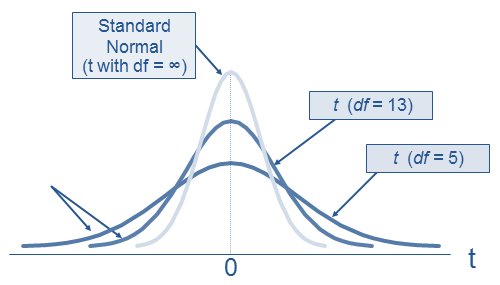# Student’s t Distribution

Student’s t distribution, or simply called t-distribution, is a form of continuous probability distributions which is formed when we are trying to estimate the mean of a population that is normally distributed, but we have a small sample size and we don’t know the population standard deviation. When we say that the sample size is small, we mean a sample size of less than 30.

Consider a random sample of n observations with mean  and standard deviation σ, from a normally distributed population with mean μ. The t-statistic is calculated as follows:The variable t follows a Student’s t distribution with (n-1) degrees of freedom. Degrees of freedom refer to the number of observations that are free to vary after sample mean has been calculated.

The t-distribution is symmetric and bell-shaped just like a normal distribution. However, it has heavier tails, which means more observations are far from the mean.

The t-distribution has a degree of freedom equal to n-1. The larger the degree of freedom, the closer is the t-distribution to normal distribution (z-statistic).

The following chart depicts t-distribution with varying degrees of freedom (df).Get our Data Science for Finance Bundle for just $29$51.